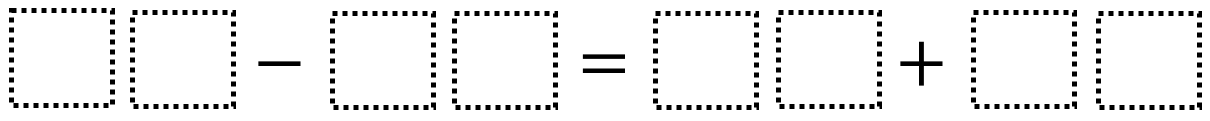# Adding and Subtracting Two-Digit Whole Numbers

Directions: Directions: Use the digits 0 to 9, at most one time each, to make a true statement.### Hint

What’s your best first move? Does it make more sense to start with the addition or subtraction? What’s the greatest value you can find? smallest value?

24+35=78-19
13+56=97-28
47+36=98-15

Source: Brian Errey

## Time Twister

Directions: Using the digits 0 to 9, at most one time each, create three different …

1.90-40=50+0

•using 0 more than once….is that allowed in this problem?

2.80-10=60+10

•using 0 more than once….is that allowed in this problem?

3.You can only use 0 one time.

4.55-12=43+0

51+32=83-0

21+11=32+0

5.60-40=10+10

6.60-10=25+25

7.9-3=3+3

8.90-30=30+30

9.55-12=43+0

10.11.78-40=23+15

12.13.Markel Brown Jr

30+30= 50=10

•Markel Brown Jr.

It is suppose to be 30+30 = 50+10

14.Danitza Marenco

It is cause it is 30+30=50+10

15.Daniel Salazar Arias

30-20=1+9

16.93-13=44+36

17.19+12=43-12

18.karine chapdelaine

87-23=49+15

19.20.45-20=16+9

21.•90-80=10+9

22.Zay'zjon Harris

50-25=17+8

23.Gerald Anderson

24.25.26.60 – 10 = 25 + 25

27.32-10=11+11

28.98-32=54+10
89-14=63+12

29.78-54=01+23

30.96 – 32 = 64
50 + 14 =64

31.35 + 45 = 80 + 10

32.90-40 = 25+25

33.9-3 = 5 + 1

34.Gregory Lancaster

60-20=10+30

35.78-40=23+15
98-32=56+10
89-14=63+12

•98-10=63+25

36.Samantha Bumpass

98 – 74 = 23 + 01

37.98-10=63+25

38.Hi, I´m a teacher. This website is so cool 🙂

39.98 – 52=36 +10 (Ivy)

69 – 08 = 24 + 37 (Ali)

97 – 06 = 73 +18 (Mrs Hamer)

80 – 36 = 19 + 25 (Luka)

98 – 16 = 50 + 32 (Aadarsh)

85 – 14 = 69 + 02 (Bayrin)

89 – 13 = 26 + 50 (Ivy)

40.I agree that, as written, using zero repeatedly is not allowed. However, allowing just zero to be used more than once might be a nice way for some kids to gain access. Then you could remove that allowance as a constraint to push kids’ thinking…

41.98-15=63+20